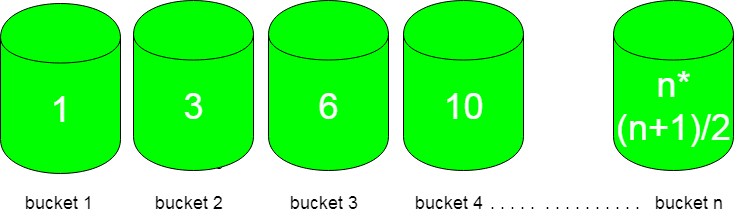Related Articles
Smallest triangular number larger than p
• Last Updated : 31 Oct, 2018

Given n number of buckets and each bucket is numbered from 1 to n and flowers in it are equal to triangular numbers. You have to choose the bucket which is left with minimum flower after picking ‘p’ flowers from it.First bucket contains only 1 flower, second bucket contains 3, third bucket contains 6 and so on following a pattern of n(n+1)/2.

Examples :

```Input : p = 4
Output : bucket 3
Explanation :
Buckets with flowers : 1 3 6 10 ....
So, bucket 3 is left with only two flowers after
selecting p flowers from it which is minimum.

Input : p = 10
Output : bucket 4
Explanation :
Bucket with flowers : 1 3 6 10 15 ...
So, selecting 10 flowers from 4th bucket leave
it with 0 flowers.
```

## Recommended: Please try your approach on {IDE} first, before moving on to the solution.

Approach :
Observing the input/output of different cases, bucket number can be calculated using formula :

```n = ceil( (sqrt(8*p+1)-1)/2 ) ;
```

How does it work?

We need smallest n such than n*(n+1)/2 >= p

So we need to find roots of equation n2 + n – 2*p >= 0.

By applying the formula discussed here, we get n = ceil( (sqrt(8*p+1)-1)/2 )

## C++

 `// CPP code to find the bucket to choose  ` `// for picking flowers out of it ` `#include ` `using` `namespace` `std; ` ` `  `int` `findBucketNo(``int` `p) ` `{ ` `   ``return` `ceil``( ( ``sqrt``( 8*p + 1 ) -1 ) / 2 ) ; ` `} ` ` `  `// Driver code ` `int` `main() ` `{ ` `  ``int` `p = 10 ;     ` `  ``cout << findBucketNo(p); ` `  ``return` `0; ` `}  `

## Java

 `//Java code to find the bucket to choose  ` `// for picking flowers out of it ` `import` `java.lang.System.*; ` ` `  `class` `GFG { ` `     `  `    ``static` `int` `findBucketNo(``int` `p) ` `    ``{ ` `        ``return` `(``int``)Math.ceil((  ` `        ``Math.sqrt( ``8``*p + ``1` `) -``1` `) / ``2` `) ; ` `    ``} ` `     `  `    ``// Driver code ` `    ``public` `static` `void` `main(String[] args) ` `    ``{ ` `        ``int` `p = ``10` `;  ` `         `  `        ``System.out.println(findBucketNo(p)); ` `    ``} ` `} ` ` `  `// This code is contributed by ` `// Smitha Dinesh Semwal `

## Python 3

 `# Python 3 code to find the bucket  ` `# to choose for picking flowers ` `# out of it ` `import` `math ` ` `  `def` `findBucketNo(p): ` ` `  `    ``return` `math.ceil( ( math.sqrt( ` `               ``8``*``p ``+` `1` `) ``-``1` `) ``/` `2` `)  ` ` `  `# Driver code ` `p ``=` `10` ` `  `print``(findBucketNo(p)) ` ` `  `# This code is contributed by ` `# Smitha Dinesh Semwal `

## C#

 `// C# code to find the bucket to choose  ` `// for picking flowers out of it ` `using` `System; ` ` `  `class` `GFG { ` `     `  `    ``static` `int` `findBucketNo(``int` `p) ` `    ``{ ` `        ``return` `(``int``)Math.Ceiling((  ` `        ``Math.Sqrt( 8*p + 1 ) -1 ) / 2 ); ` `    ``} ` `     `  `    ``// Driver code ` `    ``static` `public` `void` `Main () ` `    ``{ ` `        ``int` `p = 10 ;  ` `        ``Console.WriteLine(findBucketNo(p)); ` `    ``} ` `} ` ` `  `// This code is contributed by Ajit. `

## PHP

 ` `

Output :

```4
```

Time Complexity : O(1)

Attention reader! Don’t stop learning now. Get hold of all the important DSA concepts with the DSA Self Paced Course at a student-friendly price and become industry ready.

My Personal Notes arrow_drop_up
Recommended Articles
Page :